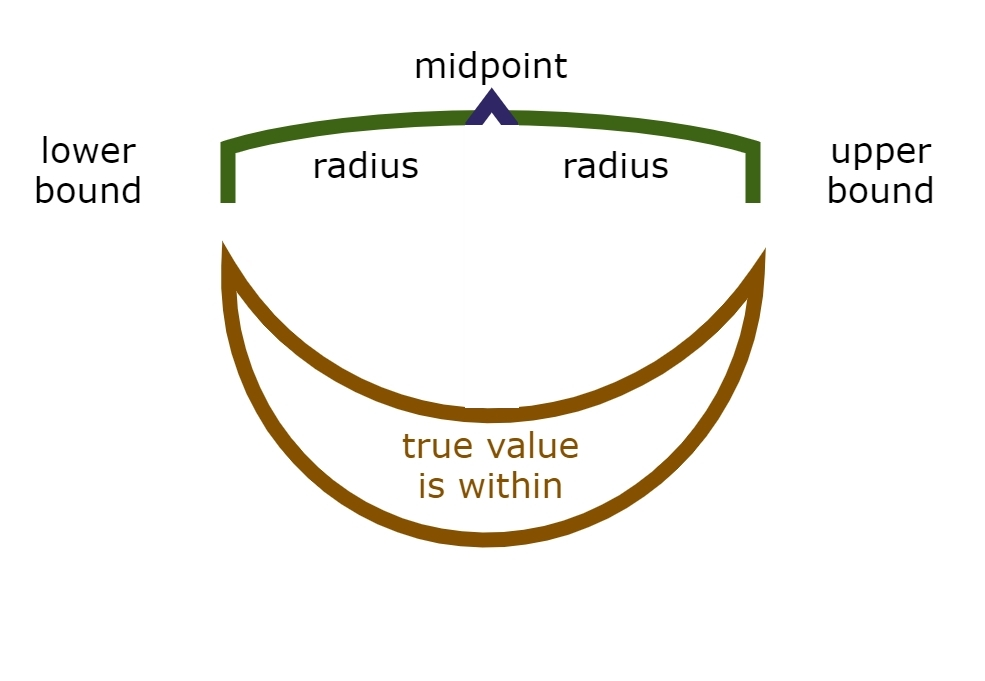There are three core types: ArbFloat, ArbReal, ArbComplex. Vectors of elements of these types are formed and used in the usual ways. Some matrix operations are supported through the underlying C library, which requires data be given in row-dominant order, we have Matrix types: ArbFloatMatrix, ArbRealMatrix, ArbComplexMatrix. Their use is described in the section on Matrices.

## ArbFloat

An ArbFloat value is an extended precision floating point value where the precision is fixed at construction.

## ArbReal

An ArbReal value is implemented in the underlying C library as an ArbFloat midpoint and a value enclosing radius.## ArbComplex

An ArbComplex value is implemented in as two ArbReal one for the real part and one for the imaginary part.

You can form an ArbComplex using two ArbReals or two ArbFloats or one of each.

• The real and imaginary parts are recovered using real(z) and imag(z) where z is an ArbComplex.

• angle(z) and magnitude(z) recover the phase angle and the lineal extent of an ArbComplex number.

• conj(z) obtains the complex conjugate of an ArbComplex number.